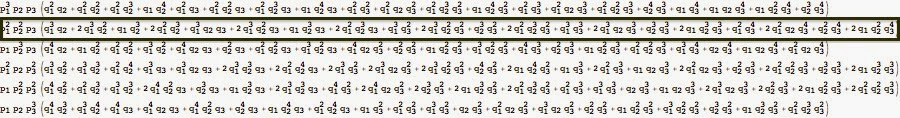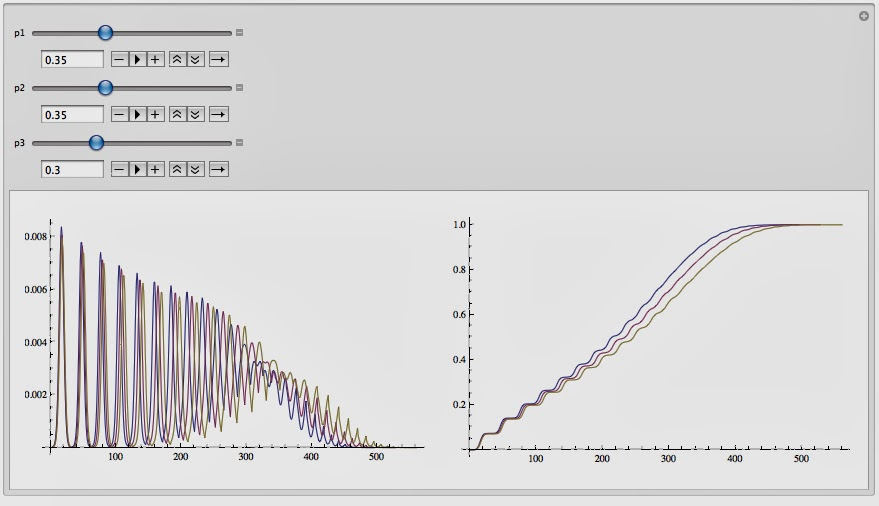Friday, March 28, 2014

Coefficients of Waiting Time Polynomials: a nice representation

I was doing some simulation for the paper I'm writing about the waiting time polynomials, and I got something unexpected.
If we sort in lexicographic order all the polynomials generated for a given length of the events vector, and we consider the coefficients, what  you get is an apparently irregular series of integers.
To capture some regularity, I decided to plot such matrix:The matrix plot, obtained considering an alphabet of three words and vectors having length=35.

Isn't nice?
Sometimes, an appropriate graphical representation helps in capture interesting aspects of your data

Stay Tuned
Cristian

Tuesday, March 18, 2014

Waiting Time Polynomials Document Classifier - Part III

Abstract
In the post is presented an innovative definition of polynomials associated to waiting time processes (analyzed in the former posts). Such polynomials are here  successfully used as document classifier. Comparative tests with SVM show significant accuracy improvements.Boolean Classification tests based on test set of 8k randomly generated documents composed using an alphabet of three words.
Introduction
To encourage who found quite intricate the formula I presented a couple of posts ago, I'm going to present you an its practical application that might be a good incentive to analyze the formal aspects with more attention :)
What I show to you today is one of several application of such approach: a document classifier having higher accuracy than traditional methods as SVM (trained with gaussian kernel) and Neural Networks back propagated.

Characteristics of the classifier
• It's a supervised learning algorithm
• It's completely non parametric
• It can be used natively to classify multiple classes datasets.

The Algorithm
Let's assume to have a training set composed by two classes of documents: Cl_1, Cl_2.

Learning Phase: Estimation of Geometrically distributed Random Variables.
1. Define an alphabet of three words {w1,w2,w3} using frequencies criteria or more sophisticated techniques.
2. For each class of training set:
• estimate parameters {p1, p2, p3} of the respective {X1(w1),X2(w2),X3(w3)} geometrically distributed random variables.
3. Calculate the polynomials associated to {X1(w1),X2(w2),X3(w3)} using:

Testing Phase: document classification
1.  for each document Di of the test set:
• Identify the number of occurrences of  {w1,w2,w3}: {O_w1,O_w2,O_w3}
• Select the polynomial for which:
•  {O_w1,O_w2,O_w3} =p1^O_w1 p2^O_w2 p3^O_w3.
• Calculate the value of the polynomials P_Cl_1, P_Cl_2 using:
1. {p1, p2, p3} estimated for Cl_1
2. {p1, p2, p3} estimated for Cl_2
2. Classify the document:
1. If (P_Cl_1>P_Cl_2) Di belongs to Cl_1
2. Else Di belongs to Cl_2
Examples
1. How to select the polynomial

Let's consider the polynomials calculated using the aforementioned formula and assume that in document Di the word w1 is repeated 2 times, the word w2 is repeated 2 times and w3 is repeated 1 time.
Then, In the step 1 of the testing phase we have to choose the polynomial boxed in the below list:Polynomials generated for O_w1+ O_w2+O_w3=5
2. Same polynomial, different values of  p1, p2, p3.

- How many polynomials are generated for O_w1+ O_w2+O_w3 = 25?
The answer is straightforward: 276 that is, all the possible configurations of 3 addends for which the sum =25. In the Encyclopedia of Integer Sequences, there are exciting explanation on such series.

- How does the polynomial change for different values of p1, p2, p3?
It's quite impressive how two polynomials can be different despite the setting of {p1, p2, p3} is almost identical.
Look at this example for which:
• On the left chart I plotted a polynomial with p1= 0.33, p2= 0.33, p3=0.34.
• On the right chart I plotted a polynomial with p1= 0.4, p2= 0.3, p3=0.3.
• In the first case the polynomial takes the maximum value for O_w1=8, O_w2= 8, O_w3 =9 ...not a big surprise!
• In the second case the polynomial takes the maximum value for O_w1=15, O_w2= 9, O_w3 =1. In this case the result is more complicated to be explained!
Document classification comparative test

To test the accuracy of my method I performed a comparative test using a randomly generated document set.
• The training set was composed by 100 documents (used to train a Gaussian Kernel SVM and to estimate params p_i for my method).
• The test set was composed by:
• Cl_1: 4000 documents randomly generated using a configuration of {p1, p2, p3}.
• Cl_2: 4000 documents randomly generated using a different configuration of {p1, p2, p3}.
• The accuracy has been tested using different configurations of {p1, p2, p3}, and considering different size of documents.
Results
First experiment:
just the first 25 words have been considered  to estimate the params {p1, p2, p3}, to train the SVM and to test the the accuracy.Accuracy results considering just the first 25 words.
Second experiment:
Same test as above using the first 35 words of the documents.Accuracy results considering just the first 35 words.
All the results showed there are referred to accuracy achieved on average using 10 different randomly generated test set and trying to configure SVM params to maximize the accuracy.
Considerations
As you can see the method based on definition of "Waiting Time Polynomials" that I'm proposing, performs significantly better than SVM.
More comparative tests will be shown in the publication I'm writing on such topic.

Further notes
Processes based on waiting time or geometrically distributed random variables are extremely important for risk assessment and risk evaluation.
I'll show you in another post some application of such polynomials in this field.
As usual, stay tuned
cristian

Thursday, March 6, 2014

The Mind Map project: a new concept of Enterprise Knowledge Management

Abstract
A project to build an innovative Information Management tool to extract, correlate and expose unstructured information.A screen shot of one of the mindmap generated by the tool. Different colors have been used to depict sub-concepts.

The demo
Time ago I published in this blog some posts where I presented some algorithms I designed to extract relevant information from documents and more in general unstructured content (as tweets, blogs post, web pages).
I don't want spend too much words, I guess a demo better describes the idea I have in mind.
It's still a prototype, and a lot of work is still required, but in this video I hope you appreciate the idea.
In the video I tested the prototype of the application using a wikipedia page.

PS
To optimize the video, watch it on youtube with the option "quality HD".

Stay Tuned
cristian

Monday, March 3, 2014

Statistical Distribution to describe a Document - My Contribute Part II

Abstract
it's presented the second step to generalize a probabilistic density function (based on geometrical distribution) to describe a document thru the underlying stochastic process.Marginal PDF and CDF for document described by three words. In red has been depicted the distribution of a document having size = 35, in Yellow size=34, in Blue size 33.
The marginal distribution that describes the density of a document for a fixed size composed by three words is the following:Example of alpha, beta, gamma parameters determination.

Introduction
The bag of words approach and almost all the related techniques to extract features from a document are based on the manipulation of frequencies associated to the words of the document. Such methodologies tend to fail when documents are characterized exactly by the same bag of words, and by the same frequency.

The proposed approach bypasses such problem since it takes in account the waiting time of the words in a document.

From 2 variables to 3 variables
In the former post I presented the easiest case: a document depicted by two words. The generalization of the problem it's not painless process.
To understand the tricks used to tackle the problem I'm going to explain the passage from 2 to 3 variables.
...To be continued.
c.
PS: I'm back!